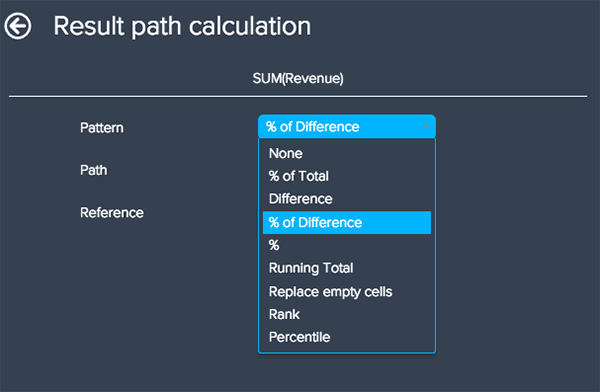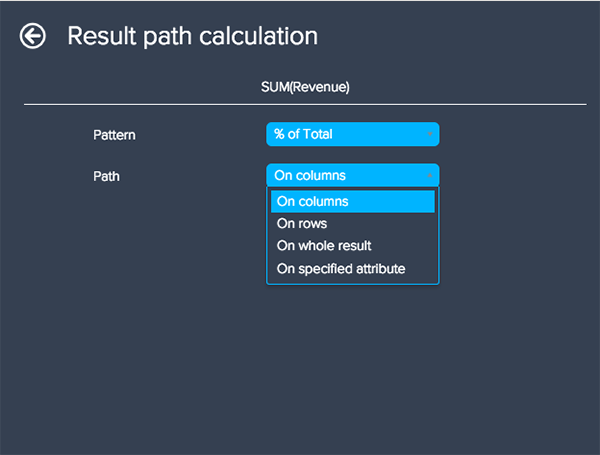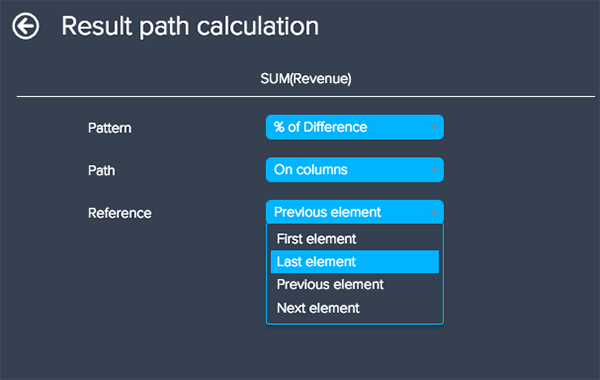Performing calculations without calculated metrics

Follow

The result path calculation is a quick and easy way to perform calculations, such as running total or the difference between results, without having to create calculated metrics.

The result path calculation will replace your original metric results with the manipulated results. If you would like to include both, you can create a new standard calculated metric with only your metric as the formula. You can then apply the result path calculation to only one metric, so your visualization will contain the original and manipulated results.

To create a new result path calculation
1. In the Result manipulation menu, select Result path calculation. All metrics currently added to Metrics will be displayed.
2. Select a pattern for your calculation. Your pattern will determine the type of calculation used. You can choose from the following options:• Percentage of total changes metric from a number to a percentage.
• Difference calculates the change in number against the chosen reference point.
• Percentage of difference calculates the change in percentage against the chosen reference point.
• Percentage displays results as the percentage of the chosen reference point.
• Running total calculates the cumulative results, by default on SUM. The aggregator can be switched to AVG, MIN, or MAX.
• Rank shows the results ranked in concurrent (1,2,2,4), dense (1,2,2,3), or single order (1,2,3,4). Concurrent and dense both group values with the same result, but concurrent will skip the next ranking number. Single will assign each value a unique ranking number.
• Percentile shows the percentile of the results across the series. This pattern is useful for tracking changes in results over time.
3. After you select a pattern, you can select your path. Your path indicates how the calculation is computed. In the example below, there are attributes on both Columns and Rows, so the path determines whether the percentage of the total shown is the share by month (Columns) or share by sales team (Rows).4. Select a reference. A reference is the result from which a calculation is computed. If you select the Replace empty cells path option, your reference can specify the result to carry forwards to the next non-empty cell.5. Click the Apply button when you are finished creating your calculation.
6. Your result path calculation will automatically be added to the Filters bar.